# AP Chemistry : Reaction Types

## Example Questions

### Example Question #21 : P H

A chemist adds 625g of solid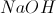to 500mL of 16M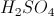. What is the pH of the solution after it reaches equilibrium?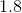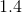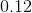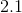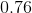Explanation:

Consider the reaction ofand: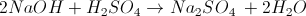Now we will calculate the moles ofin the solution prior to adding base.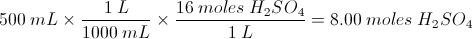We will then calculate the amount of moles ofthat react with the base.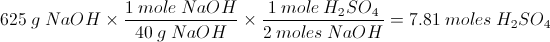We will then calculate the remaining moles of: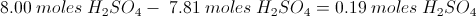We will then calculate the new concentration of sulfuric acid: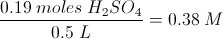Sulfuric acid is a diprotic acid, so the hydrogen ion concentration is 0.76 M.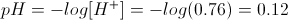### Example Question #21 : P H

If the Ksp of Mg(OH)2 is 1.2 * 10–11 and the magnesium ion concentration is 1.2 * 10–5M, at what pH does the Mg(OH)2 compound begin to precipitate?

7

11

13

4

6

11

Explanation:

The expression for Ksp is Ksp = [Mg2+][OH–]2.

Thus, [OH–] = √(1.2 * 10–11)/(1.2 * 10–5)

[OH–] = 1 * 10–3

Thus, pH = –log(1 X 10–3) = 3

pH = 14 – 3 = 11

### Example Question #29 : P H

The following is a list of acid dissociation constants for few acids.

Benzoic acid: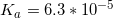Hydrofluoric acid: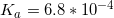Nitrous acid: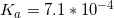Propanoic acid: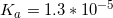One of these acids is used to create a 1M solution. It is determined that the solution has a pH of 2.44. Which of the following acids was used to make the solution?

Propanoic acid

Hydrofluoric acid

Benzoic acid

Nitrous acid

Propanoic acid

Explanation:

Since the solution has a pH of 2.44, we can find the concentration of protons in the solution using the pH equation.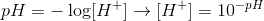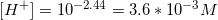All of these acids are monoprotic acids, so the equilibrium expression for each acid follows the formula for dissociation.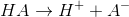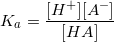The concentration for protons will be equal to the concetration of the conjugate base, since they are both in a one-to-one ratio with the acid molcule.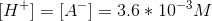Also, we can subtract the concentration of protons from the initial concentration of acid in order to find how much acid will remain at equilibrium.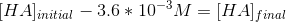Using the terms for each concentration, we can try to solve the equilibrium expression.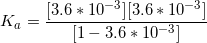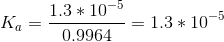Since the acid dissociation constant is equal to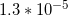, we can look at the list and determine that propanoic acid was used to make the acidic solution.

### Example Question #31 : P H

Find the pH of a 0.2M calcium hydroxide solution.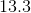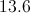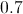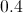Explanation:

Since every calcium hydroxide molecule will dissociate and form two hydroxide ions, the concentration of the hydroxide ions will be twice as much as the initial concentration of the base.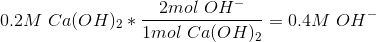This means that the concentration of hydroxide ions in the solution will be 0.4M.We can use this concentration to solve for the pOH fo the solution.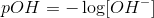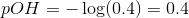Since we are looking for the pH of the solution, we simply subtract the pOH form 14.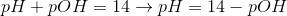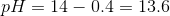This results in a pH of 13.6.

### Example Question #31 : Reactions And Equilibrium

Which of the following is a possible pH value for a basic solution?None of theseExplanation:

Basic solutions have a pH level greater than 7, which is the neutral pH. Acidic solutions have a pH level lower than 7.

### Example Question #31 : P H

Which of the following is considered a neutral pH?Explanation:

Basic solutions have a pH level greater than 7 and acidic solutions have a pH level lower than 7. A pH of 7 corresponds to a neutral solution.

### Example Question #31 : Acid Base Reactions

A chemist has an unknown solution. He think the solution is an acid. Which of the following pH's would support his hypothesis?

None of theseExplanation:

Acidic solutions have a pH level lower than 7, which is the neutral pH. Basic solutions have a pH level greater than 7.

### Example Question #31 : Acid Base Reactions

Knowing that the acid ionization constants of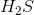are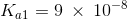and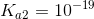, a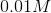solution of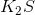will:

exhibit a neutral pH becauseis a salt

have an acidic pH

have a pH greater than 7

have a pH greater than 7

Explanation:

Potassium sulfide is soluble in water yielding the specie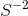which undergoes a two steps basic hydrolysis: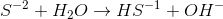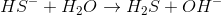The respective hydrolysis constants are: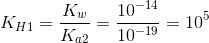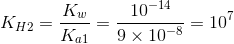Analyzing the rounded values of both hydrolysis constants, we can see that the value of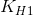is very large then we can assume the first reaction occurs to completion and controls the concentration of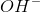in the solution which will be. Hence, the pH will be: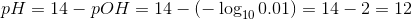### Example Question #37 : Reactions And Equilibrium

Consider the following reaction of acetic acid: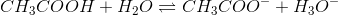The pKa for this reaction is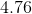. At what pH would the concentrations of the acidic and basic forms of acetic acid be equal to each other?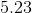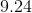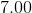Explanation:

This question is presenting us with a reversible chemical reaction of a weak acid. It provides us with the pKa of the acid, and asks us to determine where on the pH spectrum will the acidic form of acetic acid be equal to its basic form.

There is a very important concept that this question highlights, which is that a compound will always have equal amounts of its acidic and basic forms at a pH that is equal to its pKa. This can be shown mathematically by using the Henderson-Hasselbalch equation.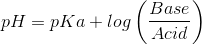As the above equation shows, when the acidic and basic forms are equal, their ratio is equal to. And the logarithm ofis equal to. Thus, what we're left with is: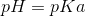### Example Question #1 : Buffers

Which of the following can be used in a buffer solution?

HNO2 and KNO2

HCl and HNO3

NaOH and KOH

NaHCO3 and K2CO3